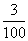# Translations of Encyclopedia about Mathematics

## Percent and Interest

Calculating percentages is an inseparable aspect of our lives: when shopping we calculate rebates and discounts, in banks we compute interest rates, and we use interest to calculate a company’s earnings.

The word percentage comes from Latin and means out of one hundred. The sign % is a mathematical symbol which expresses a percentage. Calculating with percentages has much in common with calculating with fractions except, in this case, the denominator is always 100.

For example:

3 % =